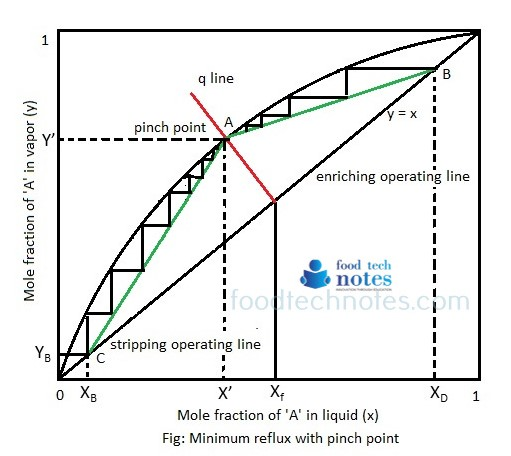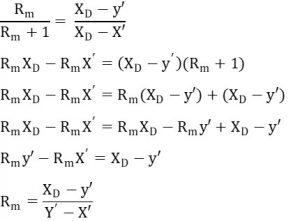# Minimum Reflux (infinite no. of plates)

At any reflux less than total, the number of plates needed for given separation is larger than total reflux. The number of plate increases continuously as reflux ratio is decreased. As the ratio becomes smaller, no. of plates required becomes very large and at definite minimum reflux ratio, number of plate become infinite.

This minimum reflux ratio can be defined as the reflux ratio (Rm) that will require infinite no. of trays for given desired separation of XD and XB. This corresponds to minimum vapor flow in tower and hence minimum reboiler and condenser sizes.

Pinch point: if R (reflux ratio) is decreased the slope of enriching operating line is decreased and intersection of this line and stripping line with ‘q’ line moves farther from 45° line and closer to equilibrium line, a “pinch point” occurs where number of steps required becomes infinite.

Thus, the intersection of an operating line and the equilibrium curve is called pinch point. A simple column will have two pinch point as there are two operating lines. The points change when operating line do. An existing column can “pinch” if its operating line is too close to equilibrium curve. This means there are several stages doing very little separation and wasting resources.

To cure pinch, the reflux and boil-up ratios can be increased to change operating lines. A pinch at the intersection of feed line and equilibrium curve indicates that column is operating at minimum reflux.We know, slope of enriching line A (x’, Y’) and B (XD, XD) is given byInvariant Zone: At pinch point in minimum reflux ratio, an acute angle is formed at the intersection of operating line and equilibrium curve, called invariant zone. In each angle an infinite no. of step is called for, representing ideal number of plates in all of which there is no change in either liquid or vapor concentration from plate to plate so Xn -1 = Xn and Yn+1 = Yn